Mathematical and Physical Journal
for High Schools
Issued by the MATFUND Foundation
 Already signed up? New to KöMaL?

# KöMaL Problems in Physics, November 2011

Show/hide problems of signs:## Problems with sign 'M'

Deadline expired on December 12, 2011.

M. 318. Measure how the visible transmittance of several piled sheets of paper depends on the number of sheets. (The intensity of light can be measured for example with a grease-spot photometer.)

(6 pont)

statistics## Problems with sign 'P'

Deadline expired on December 12, 2011.

P. 4380. At least what is the mass of the atmosphere around the Earth, if the mean radius of the Earth is 6370 km, and the average air pressure at the surface of the Earth is 100 kPa?

(3 pont)

solution (in Hungarian), statistics

P. 4381. The uniform density prism of mass M, shown in the figure is at rest on the surface of the ice of a skating rink. What is the greatest force with which it can be moved such that it does not rise? (Friction is negligible.)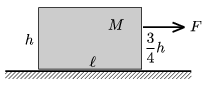(4 pont)

solution (in Hungarian), statistics

P. 4382. The bob of a 2-m long pendulum undergoes circular motion in a horizontal plane. At most how long does it take to cover a complete circle?

(4 pont)

solution (in Hungarian), statistics

P. 4383. A sonic boom is observed if the sound emitted by an airplane in a short time reaches the observer at one instant.

a) How can this happen? At what speed must the plane fly?

b) Three years ago, in November 2008, the Space Shuttle Endeavour could land only in California because of the wrong weather conditions. Interestingly the observers heard two sonic booms. How could this happen?

(5 pont)

solution (in Hungarian), statistics

P. 4384. A small ball of mass m=0.1 kg is attached to a=1 m-long thread and is hung to a horizontal peg. The small ball is at rest and another small ball of mass M=0.2 kg, is projected from the ground and collides with it, such that the collision is totally elastic and head on, and after the collision the ball at the end of the thread completes a whole circle around the peg. The distance between the peg and the ground is 2.

a) What is the least distance of x shown in the figure?

b) What is the initial velocity of the projected ball? (Speed and direction.)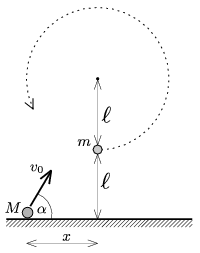(5 pont)

solution (in Hungarian), statistics

P. 4385. Water is heated in a thermally insulated container with an immersion heater. During 60% of the heating time the water, initially at a temperature of 19 oC, the water comes to the boil, and then some part of the water is boiled down, 18 g in each minute becomes steam.

a) What per cent of the water boils down?

b) What is the power of the immersion heater in watts?

(4 pont)

solution (in Hungarian), statistics

P. 4386. A sample of ideal gas of some mass is taken through the cyclic process shown in the figure. The temperature of the gas at state A is TA=200 K, and at state C is TC=1800 K.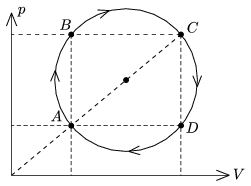a) By what factor is the pressure at state B greater than at state D?

b) What temperature belongs to the isotherm which goes through the centre of the circle?

c) Prove with calculations that the temperatures at state B and D are the same.

(3 pont)

solution (in Hungarian), statistics

P. 4387. A triangle is made from thin insulating rods of different lengths, and then the rods are charged uniformly. (The charge density along the rods is the same.) Where will the electric field strength be zero?

(6 pont)

solution (in Hungarian), statistics

P. 4388. Given the resistances of the resistors R1 and R2. Choose the resistance of the resistor R, such that the equivalent resistance of the circuit shown in the figure,

a) is also R;

b) is a given R0 value. (What values can be given for R0?)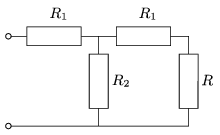(5 pont)

solution (in Hungarian), statistics

P. 4389. In order to make a flat coil, which have 200 turns, a piece of wire of negligible resistance is wound around a rectangular frame of area 50 cm2. The frame is rotated about one of the symmetry axis of the rectangle, which is in the plane of the rectangle. The frame is in magnetic field of induction 0,1 T, which is perpendicular to the axis of rotation. A bulb rated at 6,3 V, and 3 W, and a coil of resistance 10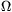, and of inductance 50 mH, are connected to the ends of the rotating coil in series.

a) What should the number of revolutions of the coil be, in order to gain 6.3 V voltage across the bulb.

b) At what phase angle does the current lag behind the voltage across the 50 mH coil?

(5 pont)

solution (in Hungarian), statistics

P. 4390. The wavelength of one of the spectral lines of a star is 591.26 nm, the corresponding wavelength in the laboratory is 592.00 nm.

a) Is the star moving towards us or away from us?

b) What is the rate of change of the distance of the star measured from us?

(4 pont)

solution (in Hungarian), statistics